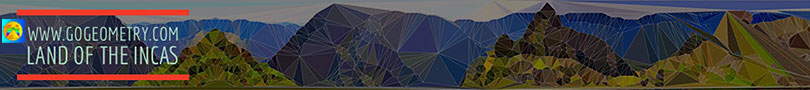Problem 114. Area of Triangles, Incircle, Excircles. Level: High School, SAT Prep, College
 In the figure below, given a triangle ABC of area S, construct the incircle with incenter I and excircles with excenters E1, E2, and E3. Let be D, E, F, G, H, and M the tangent points of triangle ABC with its excircles. If S1, S2, S3, S4, S5, and S6 are the areas of the triangles ICD, ICE, IAF, IAG, IBH, and IBM respectively, prove that S1 = S2 = S3 = S4 = S5 = S6 = S/2. View or post a solution..
 Home | Search | Problems | 111-120 | Circle Tangent Line | Email | By Antonio Gutierrez